# Coloring Sudokus

Someday you will find me
caught beneath the landslide
(Champagne Supernova, Oasis)

I recently read a book called Snowflake Seashell Star: Colouring Adventures in Numberland by Alex Bellos and Edmund Harris which is full of mathematical patterns to be coloured. All images are truly appealing and cause attraction to anyone who look at them, independently of their age, gender, education or political orientation. This book demonstrates how maths are an astonishing way to reach beauty.

One of my favourite patterns are tridokus, a sophisticated colored version of sudokus. Coloring a sudoku is simple: once that is solved it is enough to assign a color to each number (from 1 to 9).  If you superimpose three colored sudokus with no cells at the same position sharing the same color, and using again nine colors, the resulting image is a tridoku: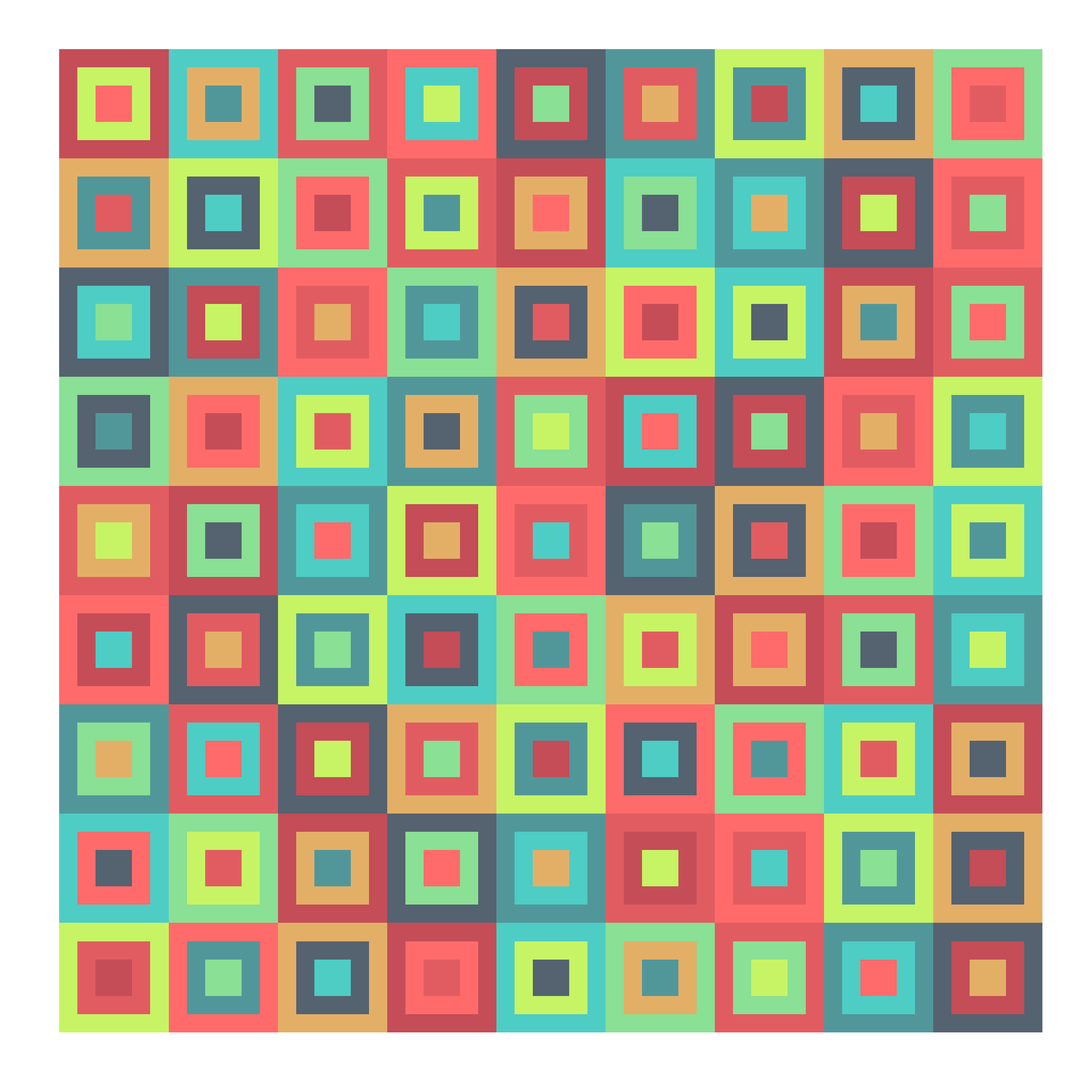There is something attractive in a tridoku due to the balance of colors but also they seem a quite messy: they are a charmingly unbalanced.  I wrote a script to generalize the concept to n-dokus. The idea is the same: superimpose n sudokus without cells sharing color and position (I call them disjoint sudokus) using just nine different colors. I did’n’t prove it, but I think the maximum amount of sudokus can be overimposed with these constrains is 9. This is a complete series from 1-doku to 9-doku (click on any image to enlarge):

I am a big fan of `colourlovers` package. These tridokus are colored with some of my favourite palettes from there:

Just two technical things to highlight:

• There is a package called sudoku that generates sudokus (of course!). I use it to obtain the first solved sudoku which forms the base.
• Subsequent sudokus are obtained from this one doing two operations: interchanging groups of columns first (there are three groups: columns 1 to 3, 4 to 6 and 7 to 9) and interchanging columns within each group then.

You can find the code here: do you own colored n-dokus!

# The Pleasing Ratio Project

Music is a world within itself, with a language we all understand (Sir Duke, Stevie Wonder)This serious man on the left is Gustav Theodor Fechner, a German philosopher, physicist and experimental psychologist who lived between 1801 and 1887. To be honest, I don’t know almost anything of his life or work exepct one thing: he did in the 1860s a thought-provoking experiment. It seems me interesting for two important reasons: he called into question something widely established and obtained experimental data by himself.

Fechner’s experiment was simple: he presented just ten rectangles to 82 students. Then he asked each of them to choose the most pleasing one and obtained revealing discoveries I will not explain here since would cause bias in my experiment. You can find more information about the original experiment here.

I have done a project inspired in Fechner’s one that I called The Pleasing Ratio Project. Once you enter in the App, you will see two rectangles. Both of them have the same area. They only vary in their length-to-width ratios. Then you will be asked to select the one that seems you most pleasing. You can do it as many times as you want (all responses are completely anonymous). Every game will confront a couple of ratios, which can vary from 1 to 3,5. In the Results section you will find the  percentage of winning games for each ratio. The one with the highest percentage will be named officially as The Most Pleasing Ratio of the World in the future by myself.

Although my experiment is absolutely inspired in Fechner’s one, there is a important difference: I can explore a bigger set of ratios doing an A/B test. This makes this one a bit richer.

The experiment has also some interesting technical features:

• the use of `shinydashboard` package to arrange the App
• the use of `shinyjs` package to add javaScript to refresh the page when use choose to play again
• to save votes in a text file
• to read it to visualize results

Will I obtain the same results as Fechner? This is a living project whose results will change over the time so you can check it regularly.

The code of the project is available in GitHub. Thanks a lot for your collaboration!

A technique succeeds in mathematical physics, not by a clever trick, or a happy accident, but because it expresses some aspect of physical truth (O. G. Sutton)

Imagine three unbalanced coins:

• Coin 1: Probability of head=0.495 and probability of tail=0.505
• Coin 2: Probability of head=0.745 and probability of tail=0.255
• Coin 3: Probability of head=0.095 and probability of tail=0.905

Now let’s define two games using these coins:

• Game A: You toss coin 1 and if it comes up head you receive 1€ but if not, you lose 1€
• Game B: If your present capital is a multiple of 3, you toss coin 2. If not, you toss coin 3. In both cases, you receive 1€ if coin comes up head and lose 1€ if not.

Played separately, both games are quite unfavorable. Now let’s define Game A+B in which you toss a balanced coin and if it comes up head, you play Game A and play Game B otherwise. In other words, in Game A+B you decide between playing Game A or Game B randomly.

Starting with 0€, it is easy to simulate the three games along 500 plays. This is an example of one of these simulations:Resulting profit of Game A+B after 500 plays  is +52€ and is -9€ and -3€ for Games A and B respectively. Let’s do some more simulations (I removed legends and titles but colors of games are the same):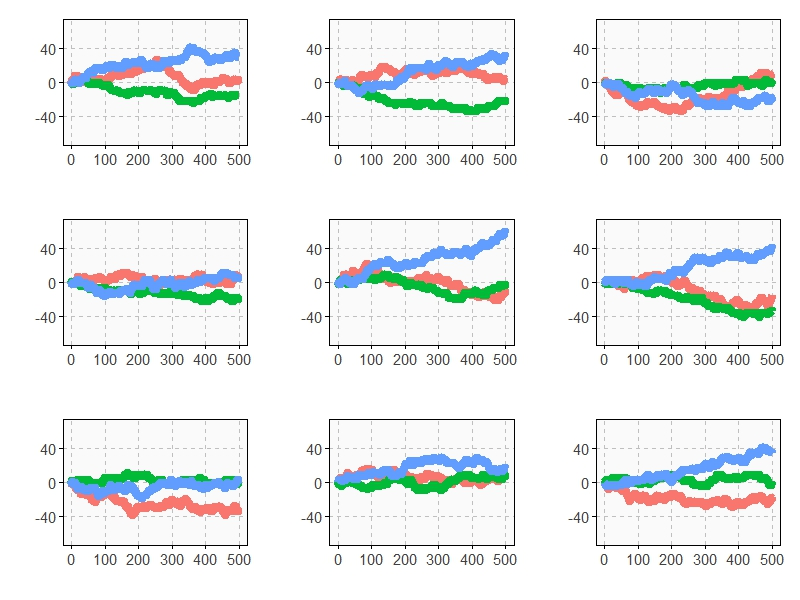As you can see, Game A+B is the most profitable in almost all the previous simulations. Coincidence? Not at all. This is a consequence of the stunning Parrondo’s Paradox which states that two losing games can combine into a winning one.

If you still don’t believe in this brain-crashing paradox, following you can see the empirical distributions of final profits of three games after 1.000 plays:After 1000 plays, mean profit of Game A is -13€, is -7€ for Game B and 17€ for Game A+B.

This paradox was discovered in the last nineties by the Spanish physicist Juan Parrondo and can help to explain, among other things, why investing in losing shares can result in obtaining big profits. Amazing:

```require(ggplot2)
require(scales)
library(gridExtra)
opts=theme(
legend.position = "bottom",
legend.background = element_rect(colour = "black"),
panel.background = element_rect(fill="gray98"),
panel.border = element_rect(colour="black", fill=NA),
axis.line = element_line(size = 0.5, colour = "black"),
axis.ticks = element_line(colour="black"),
panel.grid.major = element_line(colour="gray75", linetype = 2),
panel.grid.minor = element_blank(),
axis.text.y = element_text(colour="gray25", size=15),
axis.text.x = element_text(colour="gray25", size=15),
text = element_text(size=20),
plot.title = element_text(size = 35))
PlayGameA = function(profit, x, c) {if (runif(1) < c-x) profit+1 else profit-1}
PlayGameB = function(profit, x1, c1, x2, c2) {if (profit%%3>0) PlayGameA(profit, x=x1, c=c1) else PlayGameA(profit, x=x2, c=c2)}
####################################################################
#EVOLUTION
####################################################################
noplays=500
alpha=0.005
profit0=0
results=data.frame(Play=0, ProfitA=profit0, ProfitB=profit0, ProfitAB=profit0)
for (i in 1:noplays) {results=rbind(results, c(i,
PlayGameA(profit=results[results\$Play==(i-1),2], x =alpha, c =0.5),
PlayGameB(profit=results[results\$Play==(i-1),3], x1=alpha, c1=0.75, x2=alpha, c2=0.1),
if (runif(1)<0.5) PlayGameA(profit=results[results\$Play==(i-1),4], x =alpha, c =0.5) else PlayGameB(profit=results[results\$Play==(i-1),4], x1=alpha, c1=0.75, x2=alpha, c2=0.1)
))}
results=rbind(data.frame(Play=results\$Play, Game="A",   Profit=results\$ProfitA),
data.frame(Play=results\$Play, Game="B",   Profit=results\$ProfitB),
data.frame(Play=results\$Play, Game="A+B", Profit=results\$ProfitAB))
ggplot(results, aes(Profit, x=Play, y=Profit, color = Game)) +
scale_x_continuous(limits=c(0,noplays), "Plays")+
scale_y_continuous(limits=c(-75,75), expand = c(0, 0), "Profit")+
labs(title="Evolution of profit games along 500 plays")+
geom_line(size=3)+opts
####################################################################
#DISTRIBUTION
####################################################################
noplays=1000
alpha=0.005
profit0=0
results2=data.frame(Play=numeric(0), ProfitA=numeric(0), ProfitB=numeric(0), ProfitAB=numeric(0))
for (j in 1:100) {results=data.frame(Play=0, ProfitA=profit0, ProfitB=profit0, ProfitAB=profit0)
for (i in 1:noplays) {results=rbind(results, c(i,
PlayGameA(profit=results[results\$Play==(i-1),2], x =alpha, c =0.5),
PlayGameB(profit=results[results\$Play==(i-1),3], x1=alpha, c1=0.75, x2=alpha, c2=0.1),
if (runif(1)<0.5) PlayGameA(profit=results[results\$Play==(i-1),4], x =alpha, c =0.5)
else PlayGameB(profit=results[results\$Play==(i-1),4], x1=alpha, c1=0.75, x2=alpha, c2=0.1)))}
results2=rbind(results2, results[results\$Play==noplays, ])}
results2=rbind(data.frame(Game="A", Profit=results2\$ProfitA),
data.frame(Game="B", Profit=results2\$ProfitB),
data.frame(Game="A+B", Profit=results2\$ProfitAB))
ggplot(results2, aes(Profit, fill = Game)) +
scale_x_continuous(limits=c(-150,150), "Profit")+
scale_y_continuous(limits=c(0,0.02), expand = c(0, 0), "Density", labels = percent)+
geom_density(alpha=.75)+opts
```

# Looking For Life

` `

Machines take me by surprise with great frequency (Alan Turing)

Imagine a 8×8 grid in which cells can be alive (black colored) or empty (light gray colored):As with the One-dimensional Cellular Automata, the next state of a cell is a function of the states of the cell’s nearest neighbors (the only neighbors that we consider are the eight cells that form the immediate perimeter of a cell). To evolve this community of cells over time, rules are quite simple:

• If a live cell has less than two neighbors, then it dies (loneliness)
• If a live cell has more than three neighbors, then it dies (overcrowding)
• If an dead cell has three live neighbors, then it comes to life (reproduction)
• Otherwise, a cell stays as is (stasis)

These are the rules of the famous Conway’s Game Of Life, a cellular automaton invented in the late 1960s by John Conway trying to refine the description of a cellular automaton to the simplest one that could support universal computation. In 1970 Martin Gardner described Conway’s work in his Scientific American column. Gardner’s article inspired many people around the world to experiment with Conway’s, which eventually led to the final pieces of how the Game Of Life could support universal computation in what was surely a global collaborative effort.

In this experiment I will try to find interesting objects that can be found in the Game Of Life: static (remain the same over the time), periodic (change but repeating their initial configuration in some iterations) or moving objects (travel through the grid before disappearing). Why are interesting? Because these are the kind of objects required to perform computations.

The plan is simple: I will generate some random grids and will evolve them over time a significant number of times. After doing this, I will check those grids having still some alive cells inside. Will I find there what I am looking for?

I generated 81 grids in which live cells are randomly located using binomial random variables with probabilities equal to i/80 with i from 0 (all cells empty) to 80 (all cells alive). This is a quick way to try a set of populations with a wide range of live cells. I measure % of alive cells after each iteration. I will analyze those grids which still have live cells after all iterations. This is what happens after 150 iterations: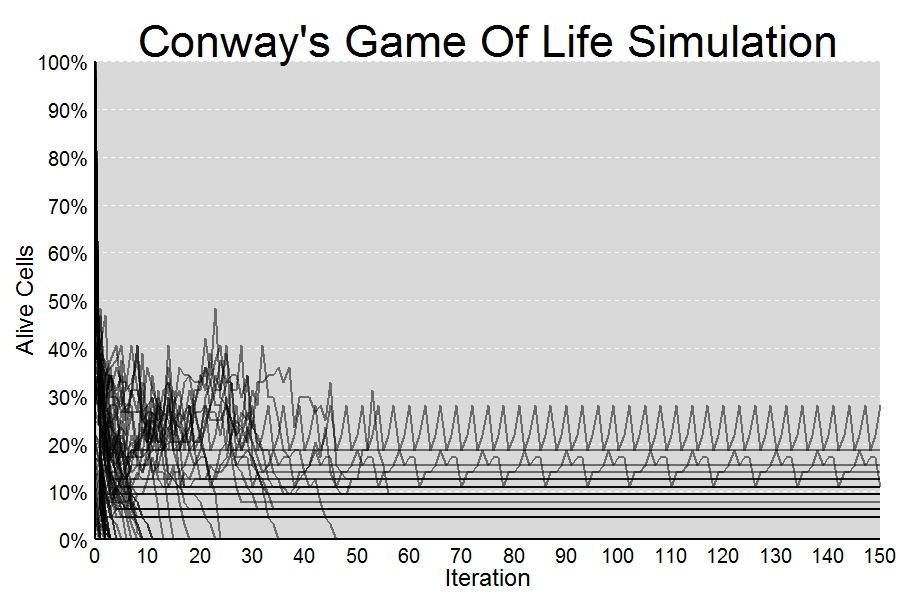I find some interesting objects. Since I keep them in my script, I can list them with `ls(pattern= "Conway", all.names = TRUE). `Two of them are specially interesting because are not static. Are those which produce non-constant lines in the previous plot.

First one is a periodic object which reproduces itself after every 3 iterations:Second one is a bit more complex. After 8 iterations appears rotated: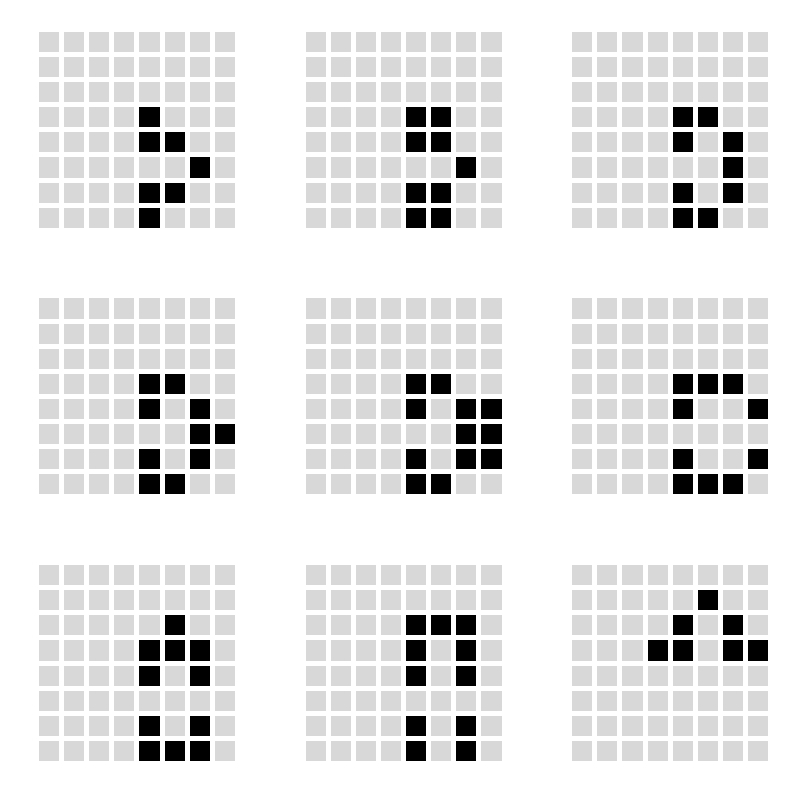What kind of calculations can be done with these objects? I don’t now yet but let’s give time to time. Do you want to look for Life?

```library(ggplot2)
library(scales)
library(gridExtra)
SumNeighbors = function (m) #Summarizes number of alive neighbors for each cell
{
rbind(m[-1,],0)+rbind(0,m[-nrow(m),])+cbind(m[,-1],0)+cbind(0,m[,-ncol(m)])+
cbind(rbind(m[-1,],0)[,-1],0)+cbind(0, rbind(0,m[-nrow(m),])[,-nrow(m)])+
cbind(0,rbind(m[-1,],0)[,-nrow(m)])+cbind(rbind(0,m[-nrow(m),])[,-1],0)
}
NextNeighborhood = function (m) #Calculates next state for each cell according to Conway's rules
{
(1-(SumNeighbors(m)<2 | SumNeighbors(m)>3)*1)-(SumNeighbors(m)==2 & m==0)*1
}
splits=80 #Number of different populations to simulate. Each population s initialized randomly
#according a binomial with probability i/splits with i from 0 to splits
iter=150
results=data.frame()
rm(list = ls(pattern = "conway")) #Remove previous solutions (don't worry!)
for (i in 0:splits)
{
z=matrix(rbinom(size=1, n=8^2, prob=i/splits), nrow=8); z0=z
results=rbind(results, c(i/splits, 0, sum(z)/(nrow(z)*ncol(z))))
for(j in 1:iter)
{z=NextNeighborhood(z); results=rbind(results, c(i/splits, j, sum(z)/(nrow(z)*ncol(z))))}
#Save interesting solutions
if (sum(z)/(nrow(z)*ncol(z))>0) assign(paste("Conway",format(i/splits, nsmall = 4), sep=""), z)
}
colnames(results)=c("start", "iter", "sparsity")
#Plot reults of simulation
opt1=theme(panel.background = element_rect(fill="gray85"),
panel.grid.minor = element_blank(),
panel.grid.major.x = element_blank(),
panel.grid.major.y = element_line(color="white", size=.5, linetype=2),
plot.title = element_text(size = 45, color="black"),
axis.title = element_text(size = 24, color="black"),
axis.text = element_text(size=20, color="black"),
axis.ticks = element_blank(),
axis.line = element_line(colour = "black", size=1))
ggplot(results, aes(iter, sparsity, group = start))+
geom_path(size=.8, alpha = 0.5, colour="black")+
scale_x_continuous("Iteration", expand = c(0, 0), limits=c(0, iter), breaks = seq(0,iter,10))+
scale_y_continuous("Alive Cells", labels = percent, expand = c(0, 0), limits=c(0, 1), breaks = seq(0, 1,.1))+
labs(title = "Conway's Game Of Life Simulation")+opt1
#List of all interesting solutions
ls(pattern= "Conway", all.names = TRUE)
#Example to plot the evolution of an interesting solution (in this case "Conway0.5500")
require(reshape)
opt=theme(legend.position="none",
panel.background = element_blank(),
panel.grid = element_blank(),
axis.ticks=element_blank(),
axis.title=element_blank(),
axis.text =element_blank())
p1=ggplot(melt(Conway0.5500), aes(x=X1, y=X2))+geom_tile(aes(fill=value), colour="white", lwd=2)+
p2=ggplot(melt(NextNeighborhood(Conway0.5500)), aes(x=X1, y=X2))+geom_tile(aes(fill=value), colour="white", lwd=2)+
p3=ggplot(melt(NextNeighborhood(NextNeighborhood(Conway0.5500))), aes(x=X1, y=X2))+geom_tile(aes(fill=value), colour="white", lwd=2)+
p4=ggplot(melt(NextNeighborhood(NextNeighborhood(NextNeighborhood(Conway0.5500)))), aes(x=X1, y=X2))+geom_tile(aes(fill=value), colour="white", lwd=2)+
#Arrange four plots in a 2x2 grid
grid.arrange(p1, p2, p3, p4, ncol=2)
```

# Batman’s Choice

A hero can be anyone, even a man doing something as simple and reassuring as putting a coat on a young boy’s shoulders to let him know the world hadn’t ended (Batman in The Dark Knight Rises)

Joker has captured Batman and keeps him into a dark and cold dungeon of Gotham City. Showing his sadistic character, Joker proposes the following game to Batman:

This is a six shooter revolver with two bullets in the cylinder. Bullets are inside two consecutive chambers. I will spin the cylinder and will fire the gun aiming to my head. If I survive you will have to do the same but you decide if you want to spin the cylinder before firing or not. If you still keep you head over your shoulders after firing, you will be free.

Joker fires and nothing happens. He survives and passes the revolver to Batman. What should Batman do? Spinning or not? What would you do?

From my point of view, answer is quite anti-intutive because the best option is not spinning the cylinder again. Spinning case is clear: probability of losing the head is 2/6=33% but what about not spinning? Doing the next shoot directly eliminates two possibilities: the previous shot of Joker and the second bullet according to direction of cylinder rotation (remember two bullets are consecutive and Joker is unfortunately  still alive). It means there is only 1 chance to dead between 4, so probability of losing the head in this scenario is 1/4=25% which is significantly lower than the first one.

Here you can find the resulting graph of simulating the game up to 500 times: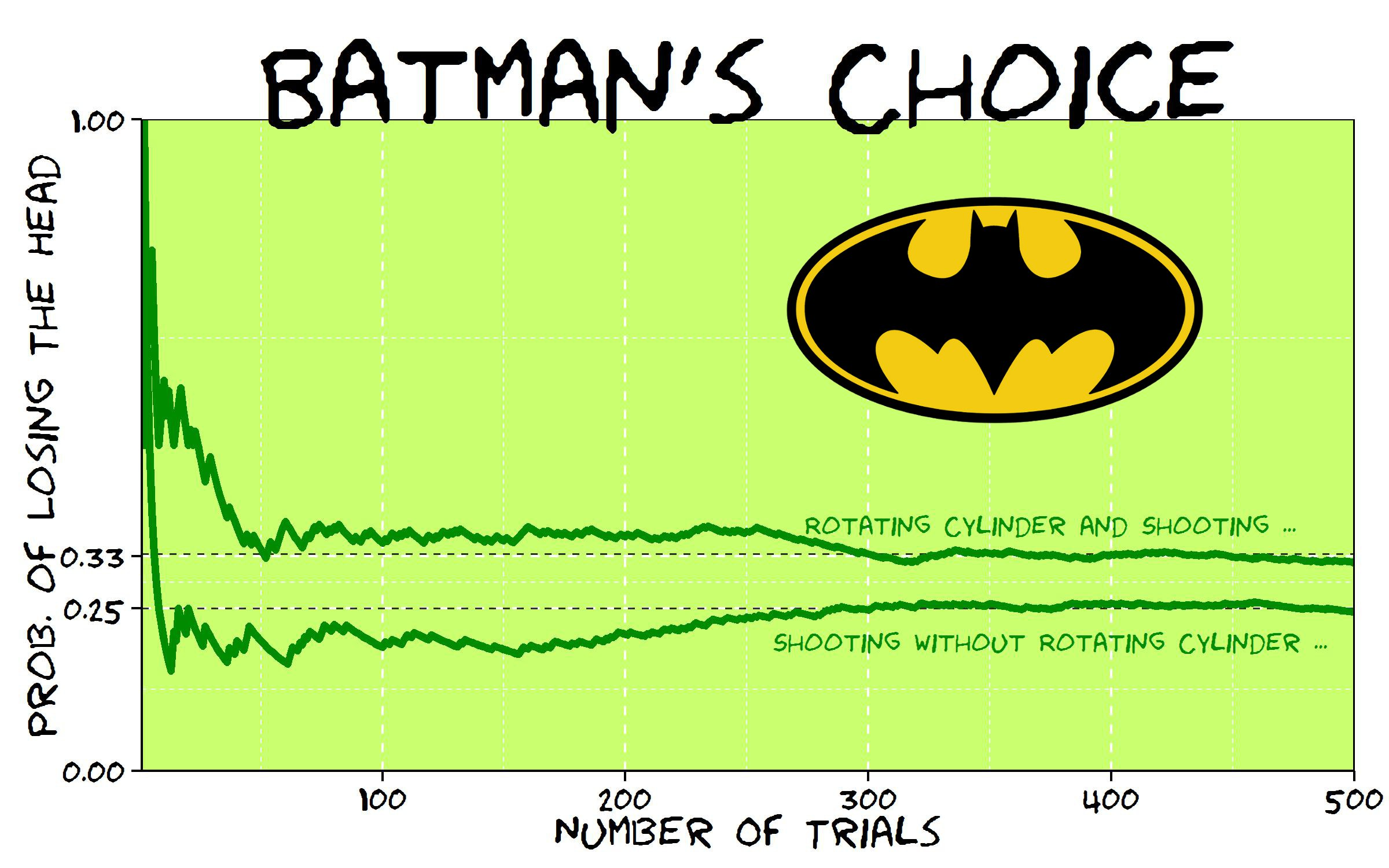Will it be the end of Batman? Not sure.

This is the code of this experiment:

```library(ggplot2)
library(extrafont)
niter=500
results=data.frame()
for (i in 1:niter)
{
bullet1=sample(1:6,1)
Joker=sample((1:6)[-c(bullet1, bullet1%%6+1)],1)
#Option 1: Shooting
Batman1=Joker%%6+1
#Option 2: Rolling and Shooting
Batman2=sample(1:6,1)
}
theme_xkcd=theme(
panel.background = element_rect(fill="darkolivegreen1"),
panel.border = element_rect(colour="black", fill=NA),
axis.line = element_line(size = 0.5, colour = "black"),
axis.ticks = element_line(colour="black"),
panel.grid = element_line(colour="white", linetype = 2),
axis.text.y = element_text(colour="black"),
axis.text.x = element_text(colour="black"),
text = element_text(size=18, family="Humor Sans"),
plot.title = element_text(size = 50)
)
p=ggplot(data=results, aes(x=iter, y=csum1))+
geom_abline(intercept = 1/4, slope = 0, size = 0.4, linetype=2, colour = "black", alpha=0.8)+
geom_abline(intercept = 1/3, slope = 0, size = 0.4, linetype=2, colour = "black", alpha=0.8)+
geom_line(aes(y=csum2), colour="green4", size=1.5, fill=NA)+
geom_line(colour="green4", size=1.5, fill=NA)+
coord_cartesian(ylim=c(.0, 1), xlim=c(1, niter))+
scale_y_continuous(breaks = c(0,round(1/4, digits = 2),round(1/3, digits = 2),1))+
geom_text(data=results[niter*.75, ], family="Humor Sans", colour="green4", y=0.38, label="Rotating Cylinder and Shooting ...", size=4, adjust=1)+
geom_text(data=results[niter*.75, ], family="Humor Sans", colour="green4", y=0.20, label="Shooting without Rotating Cylinder ...", size=4, adjust=1)+
labs(x="Number Of Trials", y="Prob. of Losing The Head", title="Batman's Choice")+
theme_xkcd
ggsave("batmans_choice.jpg", plot=p, width=8, height=5)
```

# The Three Little Pigs

The game of pig has simple rules but complex strategies. It was described for the first time in 1945  by a magician called John Scarne. Playing the pig game is easy: each turn, a player repeatedly rolls a die until either a 1 is rolled or the player decides to hold:

• If the player rolls a 1, they score nothing and it becomes the next player’s turn
• If the player rolls any other number, it is added to their turn total and the player’s turn continues
• If a player chooses to hold, their turn total is added to their score, and it becomes the next player’s turn

The first player who reach at least 100 points is the winner. For example: you obtain a 3 and then decide to roll again, obtaining a 1. Your score is zero in this turn. Next player gets the sequence 3-4-6 and decides to hold, obtaining a score of 13 points in this turn.

Despite its simplicity, the pig game has a very complex and against-intuition optimal strategy. It was calculated in 2004 by Todd W. Neller and Clifton Presser from Gettysburg College of Pennsilvania with the help of computers.

To illustrate the game, I simulated three players (pigs) playing the pig game with three different strategies:

• The Coward pig, who only rolls the die a small number of times in every turn
• The Risky pig, who rolls the die a more times than the coward one
• The Ambitious pig, who tries to obtain in every turn more points than two others

I simulated several scenarios.

• Some favorable scenarios for Coward pig:

In first scenario, the Coward pig rolls the die between 1 and 5 times each round and wins if the Risky pig asumes an excessive level of risk (rolling each time between 10 and 15 times). Trying to obtain more than the Coward is a bad option for the Ambitious pig. Simulating this scenario 100 times gives victory to Coward a 51% of times (25% to Risky and 24% to Ambitious).

Second scenario puts closer Coward and Risky pigs (first one rolls the die between 4 and 7 times  each round and second one between 6 and 9 times). Coward wins 54% of times (34% Risky and only 12% Ambitious).

Being coward seems to be a good strategy when you play against a reckless or when you are just a bit more conservative than a Risky one.

• Some favorable scenarios for Risky pig:

Rolling the die between 4 and 6 times each round seems to be a good option, even more when you are playing against a extremely conservative player who rolls no more than 3 times each time. Simulating 100 times these previous scenarios gives victory to Risky pig a 58% of times in first the case in which Coward rolls allways 1 and Risky 6 times each round (0% for Coward and only 42% form Ambitious) and 66% of times in the second one (only 5% to Coward and 29% to Ambitious).

Being Risky is a good strategy when you play against a chicken.

• Some favorable scenarios for Ambitious pig:

The Ambitious pig wins when two others turn into extremely coward and risky pigs as can be seen in the first scenario in which Ambitious wins 65% of the times (31% for Coward and 4% for Risky). Ambitious pig also wins when two others get closer and hit the die a small number of times (2 rolls the Coward and 4 rolls the Risky). In this scenario the Ambitious wins 58% of times (5% for Coward and 37% for Risky). By the way, these two scenarios sound very unreal.

Being ambitious seems to be dangerous but works well when you play against a crazy and a chicken or against very conservative players.

From my point of view, this is a good example to experiment with simulations, game strategies and xkcd style graphics.

The code:

```require(ggplot2)
require(extrafont)
#Number of hits for Coward
CowardLower=2
CowardUpper=2
#Number of hits for Risky
RiskyLower=4
RiskyUpper=4
game=data.frame(ROUND=0, part.p1=0, part.p2=0, part.p3=0, Coward=0, Risky=0, Ambitious=0)
while(max(game\$Coward)<100 & max(game\$Risky)<100 & max(game\$Ambitious)<100)
{
#Coward Little Pig
p1=sample(1:6,sample(CowardLower:CowardUpper,1), replace=TRUE)
s1=min(min(p1-1),1)*sum(p1)
#Risky Little Pig
p2=sample(1:6,sample(RiskyLower:RiskyUpper,1), replace=TRUE)
s2=min(min(p2-1),1)*sum(p2)
#Ambitious Little Pig
s3=0
repeat {
p3=sample(1:6,1)
s3=(p3+s3)*min(min(p3-1),1)
if (p3==1|s3>max(s1,s2)) break
}
game[nrow(game)+1,]=c(max(game\$ROUND)+1,s1,s2,s3,max(game\$Coward)+s1,max(game\$Risky)+s2,max(game\$Ambitious)+s3)
}
opts=theme(
panel.background = element_rect(fill="darkolivegreen1"),
panel.border = element_rect(colour="black", fill=NA),
axis.line = element_line(size = 0.5, colour = "black"),
axis.ticks = element_line(colour="black"),
panel.grid.major = element_line(colour="white", linetype = 1),
panel.grid.minor = element_blank(),
axis.text.y = element_text(colour="black"),
axis.text.x = element_text(colour="black"),
text = element_text(size=25, family="xkcd"),
legend.key = element_blank(),
legend.position = c(.2,.75),
legend.background = element_blank(),
plot.title = element_text(size = 50)
)
ggplot(game, mapping=aes(x=game\$ROUND, y=game\$Coward)) +
geom_line(color="red", size=1.5) +
geom_line(aes(x=game\$ROUND, y=game\$Risky), color="blue", size=1.5) +
geom_line(aes(x=game\$ROUND, y=game\$Ambitious), color="green4", size=1.5) +
geom_point(aes(x=game\$ROUND, y=game\$Coward, colour="c1"), size=5.5) +
geom_point(aes(x=game\$ROUND, y=game\$Risky, colour="c2"), size=5.5) +
geom_point(aes(x=game\$ROUND, y=game\$Ambitious, colour="c3"), size=5.5) +
ggtitle("THE THREE LITTLE PIGS") +
xlab("ROUND") + ylab("SCORING") +
geom_text(aes(max(game\$ROUND), max(max(game\$Coward, game\$Risky, game\$Ambitious)), hjust=1.2, family="xkcd", label="WINNER!"), size=10)+
geom_hline(yintercept=100, linetype=2, size=1)+
scale_y_continuous(breaks=seq(0, max(max(game\$Coward, game\$Risky, game\$Ambitious))+10, 10))+
scale_x_continuous(breaks=seq(0, max(game\$ROUND), 1))+
scale_colour_manual("",
labels = c(paste("Coward: ", CowardLower, "-", CowardUpper, " hits", sep = ""), paste("Risky: ", RiskyLower, "-", RiskyUpper, " hits", sep = ""), "Ambitious"),
breaks = c("c1", "c2", "c3"),
values = c("red", "blue", "green4"))+ opts
```